## Wednesday, August 29, 2012

### Power Electronics Objective Questions with Answers: Part 1

 " Six MOSFETs connected in a bridge configuration (having no other power device) MUST be operated as a Voltage Source Inverter (VSI) ". This statement is           [GATE-2007]
A. True, because being majority carrier devices, MOSFETs are voltage driven
B. True, because MOSFETs have inherently anti parallel diodes
C. False, because it can be operated both as current source Inverter (CSI) or a VSI
D. False, because MOSFETs can be operated as excellent constant current sources in the saturation region

 A single phase full - wave half controlled bridge converter feeds an inductive load. The two SCRs in the converter are connected to a common DC bus. The converter has to have a free wheeling diode  [GATE-2007]
A. because the converter inherently does not provide for free wheeling
B. Because the converter does not provide for free wheeling for high values of triggering angles
C. Or else the free wheeling action of the converter will cause shorting of the AC supply
D. Or else if a gate pulse to one of the SCRs is missed, it will subsequently cause a high load current in the other SCR

 The power electronic converter shown in the figure has a single pole double throw switch. the pole P of the switch is connected alternately to throws A and B. The converter shown is a  [GATE-2010]A. Step down chopper( buck converter)
B. Half- wave rectifier
C. Step Up chopper ( boost converter)
D. Full wave converter

 Figure shows a composite switch consisting of a power transistor (BJT) in series with a diode. Assuming that the transistor switch and the diode are ideal, the I-V characteristic of the composite switch is [GATE-2010]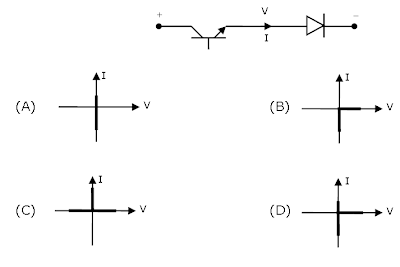The fully controlled thyristor converter in the figure is fed from a single-phase source. When the firing angle is 0°, the dc output voltage of the converter is 300 V. What will be the output voltage for a firing angle of 60°, assuming continuous conduction?  [GATE-2010]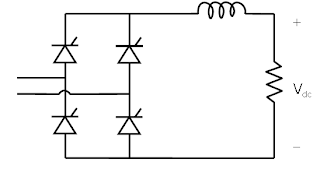(A) 150V
(B) 210V
(C) 300V
(D) 100πV

 The manufacturer of a selected diode gives the rate of fall of the diode current di/dt = 20 A/ms, and a reverse recovery time of trr =5 ms. What is value of peak reverse current?

Ans: 44.72A

The peak reverse current is given as:The storage charge QRR is calculated as Qrr = (1/2)*(di/dt)* SQU.(trr)

= 1/2 X 20A/uS X 5u X 5u = 50uC.
substitute the Qrr value in Irr equation.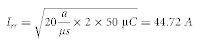A three phase current source inverter used for the speed control of an induction motor is to be realized using MOSFET switches as shown below. Switches S1 to S6 are identical switches [GATE2011]The proper configuration for realizing switches S1 to S6 isCircuit turn-off time of an SCR is defined as the time   [GATE2011]
A. Taken by the SCR to turn off
B. Required for SCR current to become zero
C. For which the SCR is reverse biased by the commutation circuit
D. For which the SCR is reverse biased to reduce its current below the holding current

 A single phase fully controlled thyristor bridge ac-dc converter is operating at a firing angle of 25 degree, and an overlap angle 10 degree with constant dc output current of 20A. The fundamental power factor ( displacement factor) at input ac mains is   [GATE2007]
A.  0.78
B.  0.827
C.  0.866
D.  0.9

 A three phase fully controlled thyristor bridge converter is used as line commutated inverter to feed 50 KW power at 420 V DC to a three phase 415V(line), 50Hz as mains. Consider Dc link current to be constant. The rms current of the thyristor is       [GATE2007]
A. 119.05A
B. 79.37A
C. 68.73A
D. 39.68A

7:56 PM

#### 12 comments:

1.very good but solution should also be explained
it would be more benifitial

1.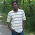Thanks for your comments. Sure we will implement your suggestion in future posts

2.please post with solutions

3.question num 8 both c and d are option

1.option C is correct as the question is circuit turn off time not device turn off time.
Option D is right for device turn off time

4.It was very usefull for my gate preparation...

5.It was very usefull for my gate preparation..

6.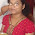thank u sir n upload more bits

7.1 que ans is A .......plz correct them

1.Thanks for reading and asking question...MOSFET is voltage driven device, majority carrier device. That is true. But here the question is when it is connected in bridge configuration what will happen?... in that case, Option D is correct. Please feel free to ask more questions

8.thank u....please post objestive questions of power system and engineering mathematics

9.Q7 and should be a